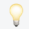This website requires JavaScript.# A general formula for Hecke-type false theta functions

Dec 2022

In recent work where Matsusaka generalizes the relationship betweenHabiro-type series and false theta functions after Hikami, five families ofHecke-type double-sums of the form \begin{equation*} \left( \sum_{r,s\ge 0}-\sum_{r,s<0}\right)(-1)^{r+s}x^ry^sq^{a\binom{r}{2}+brs+c\binom{s}{2}},\end{equation*} where $b^2-ac<0$, are decomposed into sums of products of thetafunctions and false theta functions. Here we obtain a general formula for suchdouble-sums in terms of theta functions and false theta functions, whichsubsumes the decompositions of Matsusaka. Our general formula is similar instructure to the case $b^2-ac>0$, where Mortenson and Zwegers obtain adecomposition in terms of Appell functions and theta functions.Q1论文试图解决什么问题？
Q2这是否是一个新的问题？
Q3这篇文章要验证一个什么科学假设？
0